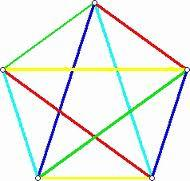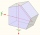# Pentagon

Calculate the length of side, circumference and area of a regular pentagon, which is inscribed in a circle with radius r = 6 cm.

a =  7.0534 cm
o =  35.2671 cm
S =  85.5951 cm2

### Step-by-step explanation:Did you find an error or inaccuracy? Feel free to write us. Thank you!Tips to related online calculators
Pythagorean theorem is the base for the right triangle calculator.

#### You need to know the following knowledge to solve this word math problem:

We encourage you to watch this tutorial video on this math problem:

## Related math problems and questions:

• 30-gonAt a regular 30-gon the radius of the inscribed circle is 15cm. Find the "a" side size, circle radius "R", circumference, and content area.
• Diagonals of pentagonCalculate the diagonal length of the regular pentagon: a) inscribed in a circle of radius 12dm; b) a circumscribed circle with a radius of 12dm.
• DecagonCalculate the area and circumference of the regular decagon when its radius of a circle circumscribing is R = 1m
• Regular n-gonWhich regular polygon have a radius of circumscribed circle r = 10 cm and the radius of inscribed circle p = 9.962 cm?
• Hexagon ACalculate area of regular hexagon inscribed in circle with radius r=9 cm.
• Hexagon in circleCalculate the radius of a circle whose length is 10 cm greater than the circumference of a regular hexagon inscribed in this circle.
• Nine-gonCalculate the perimeter of a regular nonagon (9-gon) inscribed in a circle with a radius 13 cm.
• Inscribed and described circleFind the radii of a circle inscribed and circumscribed by a regular pentagon whose side measures 3 cm.Calculate the content of a regular 15-sides polygon inscribed in a circle with radius r = 4. Express the result to two decimal places.
• Hexagon ABCDEFIn the regular hexagon ABCDEF, the diagonal AE has a length 8cm. Calculate the circumference and the hexagon area.
• n-gonWhat is the side length of the regular 5-gon inscribed in a circle of radius 12 cm?
• Regular 5-gonCalculate the area of the regular pentagon with side 7 cm.
• PentagonCalculate the area of a regular pentagon, which diagonal is u=16.
• Inscribed circleXYZ is right triangle with right angle at the vertex X that has inscribed circle with a radius 5 cm. Determine area of the triangle XYZ if XZ = 14 cm.
• Right triangleCalculate the missing side b and interior angles, perimeter, and area of ​​a right triangle if a=10 cm and hypotenuse c = 16 cm.
• Hexagonal pyramidCalculate the surface area of a regular hexagonal pyramid with a base inscribed in a circle with a radius of 8 cm and a height of 20 cm.
• NonagonCalculate the area and perimeter of a regular nonagon if its radius of the inscribed circle is r = 10cm